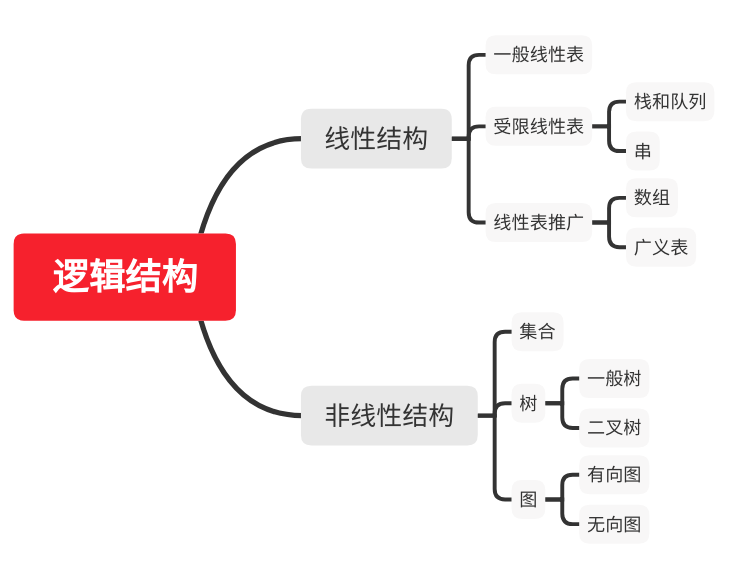## 一、数据结构基本概念

• 数据

• 数据元素

• 数据对象

• 数据类型

• 数据结构

### 2. 数据结构三要素

• 数据的逻辑结构• 数据的存储结构

1. 顺序存储
2. 链式存储
3. 索引存储
4. 散列存储（哈希存储）
• 数据的运算

## 二、算法和算法评价

• 算法概念

• 算法特性

• 有穷性

• 确定性

• 可行性

• 输入

• 输出

### 2. 算法的评价度量

• 时间复杂度

$$T(n) = O(f(n))$$
• 空间复杂度

$$S(n) = O(g(n))$$

## 三、牛刀小试

1. 已知一个算法由下列递归方程表示，求该算法的时间复杂度。
$$T(n) \begin{cases} 1,&n = 1\\ 2T(n/2) + n,&n > 1 \end{cases}$$

$$T(2^k) = 2T(2^{k-1}) + 2^k = 2^2T(2^{k-2}) + 2^22^k$$，

$$\therefore$$得出一般递推式：

$$T(2^k) = 2^kT(2^0) + k2^k = (k + 1)2^k$$，即：

$$T(n) = 2^{log_2n} + log_2n*n = n(log_2n + 1)$$

$$\therefore O(n) = nlog_2n$$

1. 计算下列程序段的时间复杂度

$$O(n) = n$$

$$O(n) = \sqrt n$$